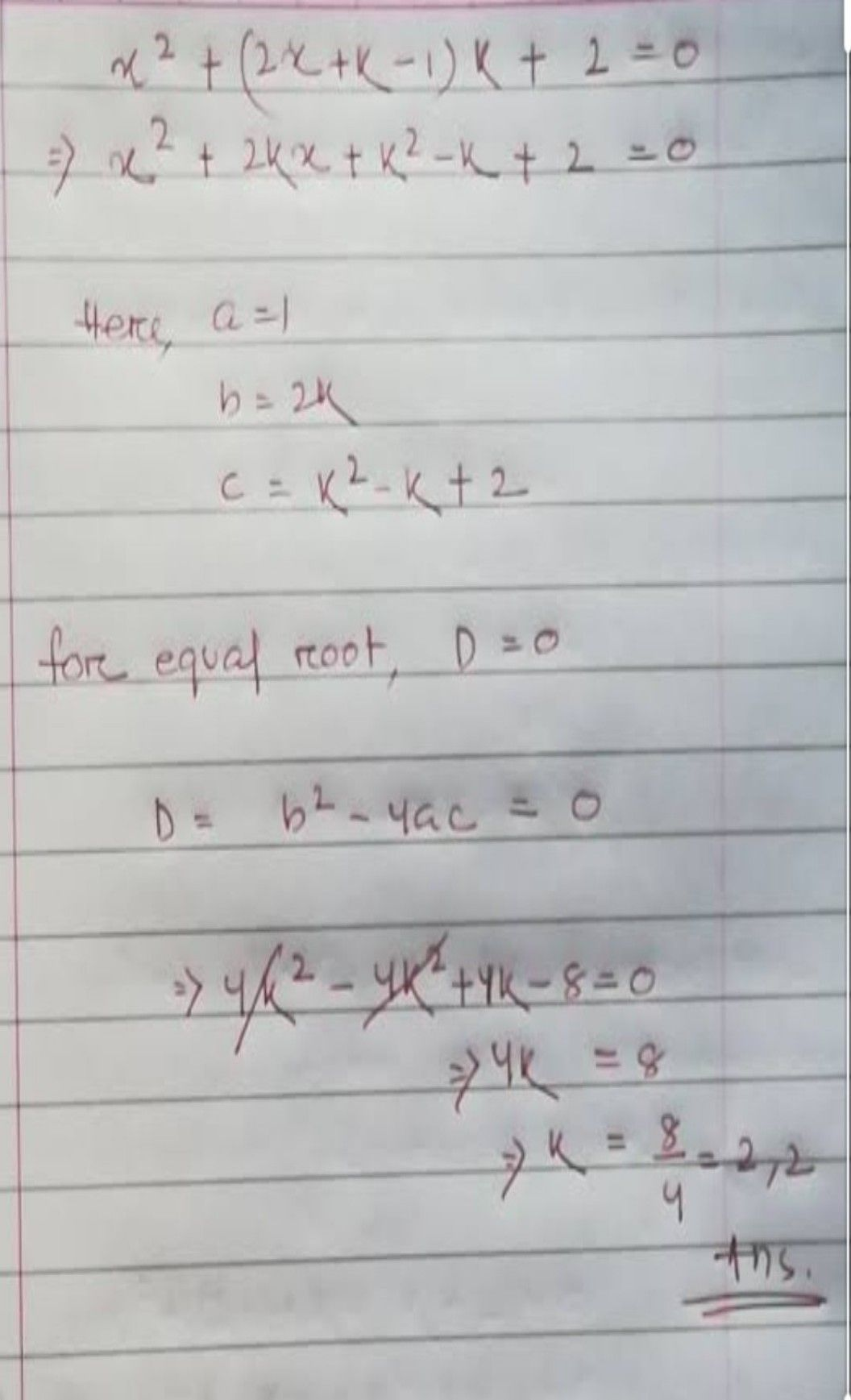Symbol
ProblemWhich equation has no real solutions? $A$ $2x^{2}+2x+15=0$ $B$ $2x^{2}+5x-3=0$ $\bar{C}$ © $x^{2}+7x+2=0$ $D$ $\times 2-4x+2=0$
10th-13th grade
Algebra
SolutionQanda teacher - nasreensk